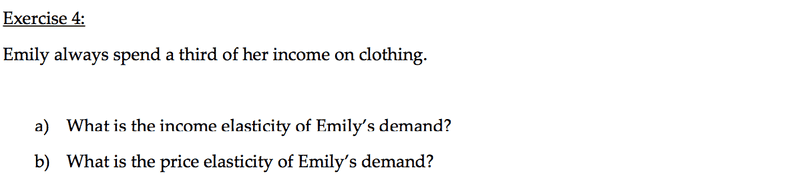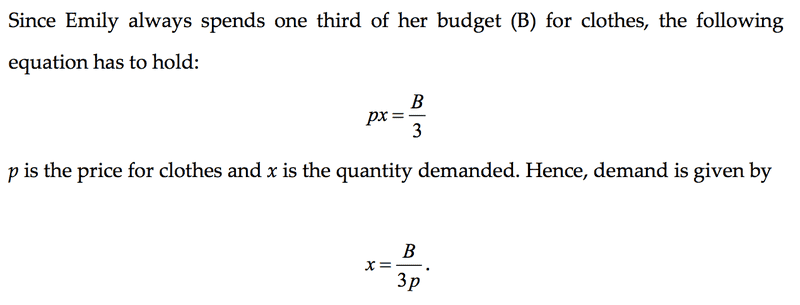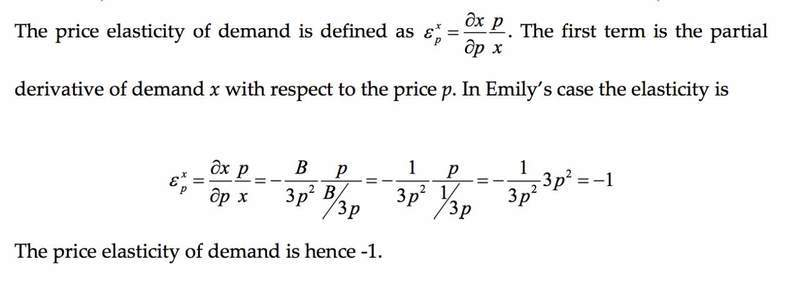# Price Elasticity, Partial Derivative

levijohnson
Hi all,

I've got a question regarding a price elasticity problem and a partial derivative.
That's what's given for the exercise:So, first of all we calculate all the demand with the given information. Which is:And then we come to the actual problem. (4. b) )How do they get the partial derivative here? I assume that they are using the quotient rule, right? I would be really glad for a step by step explanation.

Thank you so much!